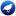bayes

This category contains 19 nodes.BayesianLogisticRegression (3.7)

Implements Bayesian Logistic Regression for both Gaussian and Laplace Priors. For more information, see Alexander Genkin, David DBayesNet (3.7)

Bayes Network learning using various search algorithms and quality measures. Base class for a Bayes Network classifierComplementNaiveBayes (3.7)

Class for building and using a Complement class Naive Bayes classifier. For more information see, Jason DDMNBtext (3.7)

Class for building and using a Discriminative Multinomial Naive Bayes classifierHNB (3.7)

Contructs Hidden Naive Bayes classification model with high classification accuracy and AUC. For more information refer to: HNaiveBayes (3.7)

Class for a Naive Bayes classifier using estimator classesNaiveBayesMultinomial (3.7)

Class for building and using a multinomial Naive Bayes classifierNaiveBayesMultinomialText (3.7)

Multinomial naive bayes for text data